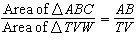Name:    Quiz 5.1-5.4

Multiple Choice
Identify the choice that best completes the statement or answers the question.

The two triangles are similar. Find the measure of the angle.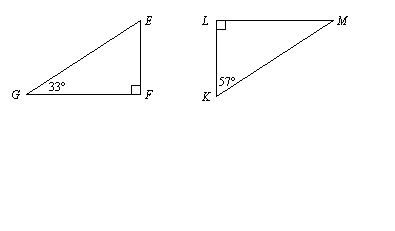1.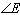a. 33° c. 57° b. 180° d. 90°

2.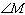a. 180° c. 90° b. 33° d. 57°

The polygons are similar. Find the value of x.

3.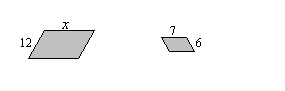a. 13 c. 12 b. 14 d. 7

4.

The ratio of the perimeters is 3:5.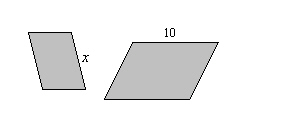a. 3 c. 4.1 b. 8 d. 6

5.

The ratio of the perimeters is 6:3.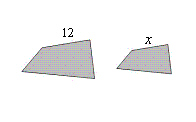a. 6 c. 7.4 b. 8 d. 3

Use the map to find the actual distance between the cities.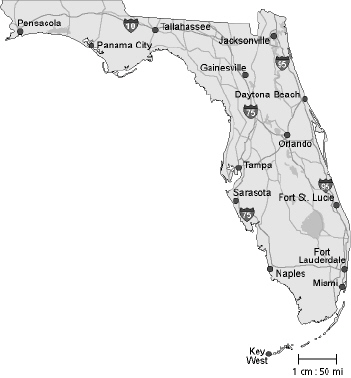6.

Pensacola and Tallahassee
 a. 350 mi c. 125 mi b. 175 mi d. 250 mi

7.

Naples and Miami
 a. 175 mi c. 100 mi b. 200 mi d. 50 mi

Numeric Response

Find the missing dimension. Use the scale factor 1:12.

1.

 Item Model Actual Courtyard Width: 2.1 ft Width: __?__ yd

1.

How are corresponding side lengths of two similar figures related?

Triangle ABC is similar to Triangle TVW. Tell whether the statement is true or false. Explain your reasoning.

2.